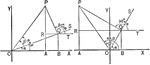### Sum of 2 Acute Angles

Illustration of two triangles, showing the sine of the sum of two acute angles expressed in terms of…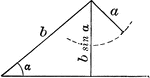### Ambiguous Case

Illustration of one possible outcome (no triangle occurs) when discussing the ambiguous case using the…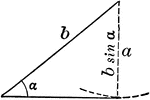### Ambiguous Case

Illustration of one possible outcome (1 triangle occurs) when discussing the ambiguous case using the…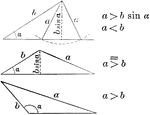### Ambiguous Case

Illustration of one possible outcome (2 triangles occur) when discussing the ambiguous case using the…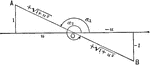### Negative Cotangent Angles

Illustration that can be used to show that if the cotangent of an angle is negative the angle must terminate…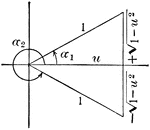### Angle Expressed As An Inverse Function

Illustration that can be used to show that when given an angle, expressed as an inverse function of…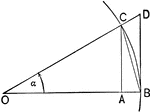### Triangles and Sectors in Quadrant I

Illustration of an angle &alpha with the vertex at the center, O, of a circle with radius OB. AC and…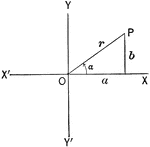Illustration of an angle &alpha with the terminal side used to draw a triangle in quadrant I.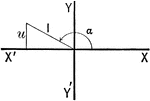Illustration of an angle with the terminal side used to draw a triangle in quadrant II.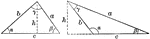### Law of Sines

Illustration two types of triangles that can be used to model the law of sines. "In a plane triangle…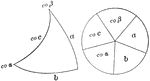### Napier's Right Spherical Triangle

Illustration of a right spherical triangle and the five circular parts placed in the sectors of a circle…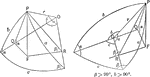### Relationships In A Spherical Triangle

Illustration used, with the law of sines, to find the relation between two sides of a spherical triangle…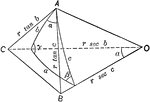### Relationships In A Spherical Triangle

Illustration used, with the law of cosines, to find the relation between the three sides and an angle…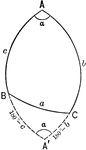### Relationships In A Spherical Triangle

Illustration used to extend the law of cosines when finding the relation between the three sides and…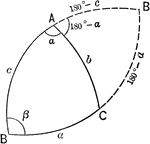### Relationships In A Spherical Triangle

Illustration used to extend the law of cosines when finding the relation between the three sides and…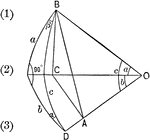### Right Spherical Triangle

Illustration of a right spherical triangle with a and b the sides, and α and β the angles…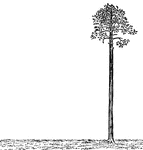### Palm Tree Perpendicular to Ground

Illustration of a palm tree that is perpendicular to the ground. The tree is perfectly straight, as…